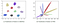# Review — AdderNet: Do We Really Need Multiplications in Deep Learning? (Image Classification)

## Using Addition Instead of Multiplication for Convolution, Lower Latency Than the Conventional CNN

• Compared with multiplications, additions are much cheaper and reduce the computation costs.

# Outline

2. Other Concerning Issues: BN, Derivatives, Learning Rate
3. Experimental Results

## 1.1. Generalized Filters

• Generally, the output feature Y indicates the similarity between the filter and the input feature:

# 2. Other Concerning Issues: BN, Derivatives, Learning Rate

## 2.1. Batch Normalization (BN)

• After addition, batch normalization (BN) is used to normalize Y to an appropriate range and all the activation functions used in conventional CNNs can then be used in the proposed AdderNets.
• Although the BN layer involves multiplications, its computational cost is significantly lower than that of the convolutional layers and can be omitted.
• (Will there be any BN just using addition in the future?)

## 2.2. Derivatives

• The derivative of l1-norm is not good for gradient descent. Thus, the derivative of l2-norm is considered:
• To prevent gradients from exploding, the gradient of X is clipped to [-1,1].
• Then the partial derivative of output features Y with respect to the input features X is calculated as:

• An adaptive learning rate for different layers in AdderNets is used:
• The local learning rate can therefore be defined as:

# 3. Experimental Results

## 3.1. MNIST

• LeNet-5-BN is trained.
• CNN achieves a 99.4% accuracy with 435K multiplications and 435K additions.
• By replacing the multiplications in convolution with additions, the proposed AdderNet achieves a 99.4% accuracy, which is the same as that of CNNs, with 870K additions and almost no multiplication.
• In fact, the theoretical latency of multiplications in CPUs is also larger than that of additions and subtractions.
• For example, in VIA Nano 2000 series, the latency of float multiplication and addition is 4 and 2, respectively. The AdderNet using LeNet-5 model will have 1.7M latency while CNN will have 2.6M latency in this CPU.

## 3.2. CIFAR

• For VGG-small model, AdderNets achieve nearly the same results (93.72% in CIFAR-10 and 72.64% in CIFAR-100) with CNNs (93.80% in CIFAR-10 and 72.73% in CIFAR-100) with no multiplication.
• Although the model size of BNN is much smaller than those of AdderNet and CNN, its accuracies are much lower (89.80% in CIFAR-10 and 65.41% in CIFAR-100).
• As for the ResNet-20, CNNs achieve the highest accuracy (i.e. 92.25% in CIFAR-10 and 68.14% in CIFAR-100) but with a large number of multiplications (41.17M).
• The proposed AdderNets achieve a 91.84% accuracy in CIFAR-10 and a 67.60% accuracy in CIFAR-100 without multiplications, which is comparable with CNNs.
• In contrast, the BNNs only achieve 84.87% and 54.14% accuracies in CIFAR-10 and CIFAR-100.
• The results in ResNet-32 also suggest that the proposed AdderNets can achieve similar results with conventional CNNs.

## 3.3. ImageNet

• AdderNet achieve a 66.8% top-1 accuracy and an 87.4% top-5 accuracy in ResNet-18, which demonstrate the adder filters can extract useful information from images.
• Although the BNN can achieve high speed-up and compression ratio, it achieves only a 51.2% top-1 accuracy and a 73.2% top-5 accuracy in ResNet-18.
• Similar results for deeper ResNet-50.

## 3.4. Visualization ResultsVisualization of features in AdderNets and CNNs. Features of CNNs in different classes are divided by their angles.
• Numbers of neurons in each convolutional layer are 32, 32, 64, 64, 128, 128, and 2, respectively.
• AdderNets utilize the l1-norm to distinguish different classes. The features tend to be clustered towards different class centers.
• The visualization results demonstrate that the proposed AdderNets could have the similar discrimination ability to classify images as CNNs.Visualization of filters in the first layer of LeNet-5-BN on MNIST
• The visualization experiments further demonstrate that the filters of AdderNets can effectively extract useful information from the input images and features.